SSC CGL Previous Year Questions: Number System and HCF & LCM- 1

# SSC CGL Previous Year Questions: Number System and HCF & LCM- 1 - SSC CGL

Test Description

## 20 Questions MCQ Test SSC CGL (Tier - 1) - Previous Year Papers (Topic Wise) - SSC CGL Previous Year Questions: Number System and HCF & LCM- 1

SSC CGL Previous Year Questions: Number System and HCF & LCM- 1 for SSC CGL 2023 is part of SSC CGL (Tier - 1) - Previous Year Papers (Topic Wise) preparation. The SSC CGL Previous Year Questions: Number System and HCF & LCM- 1 questions and answers have been prepared according to the SSC CGL exam syllabus.The SSC CGL Previous Year Questions: Number System and HCF & LCM- 1 MCQs are made for SSC CGL 2023 Exam. Find important definitions, questions, notes, meanings, examples, exercises, MCQs and online tests for SSC CGL Previous Year Questions: Number System and HCF & LCM- 1 below.
Solutions of SSC CGL Previous Year Questions: Number System and HCF & LCM- 1 questions in English are available as part of our SSC CGL (Tier - 1) - Previous Year Papers (Topic Wise) for SSC CGL & SSC CGL Previous Year Questions: Number System and HCF & LCM- 1 solutions in Hindi for SSC CGL (Tier - 1) - Previous Year Papers (Topic Wise) course. Download more important topics, notes, lectures and mock test series for SSC CGL Exam by signing up for free. Attempt SSC CGL Previous Year Questions: Number System and HCF & LCM- 1 | 20 questions in 20 minutes | Mock test for SSC CGL preparation | Free important questions MCQ to study SSC CGL (Tier - 1) - Previous Year Papers (Topic Wise) for SSC CGL Exam | Download free PDF with solutions
 1 Crore+ students have signed up on EduRev. Have you?
SSC CGL Previous Year Questions: Number System and HCF & LCM- 1 - Question 1

### If A is the smallest three digit number divisible by both 6 and 7 and B is the largest four digit number divisible by both 6 and 7, then what is the value of B – A?         (SSC MTS 2018)

Detailed Solution for SSC CGL Previous Year Questions: Number System and HCF & LCM- 1 - Question 1

The smallest three digit number divisible by 6 and 7 is = 42 × 3 = 126

⇒ A = 126

And to find the largest four digit number digit number divisible by 6 and 7, we have to divide 9999 by 42

⇒ 9999 = 42 × 238 + 3

⇒ The largest four digit number divisible by 42 is 9999 – 3 = 9996

⇒ B = 9996

So, the required difference = 9996 – 126

∴ The difference between B and A is 9870

SSC CGL Previous Year Questions: Number System and HCF & LCM- 1 - Question 2

### What is the median of the given data? 41, 43, 46, 50, 85, 61, 76, 55, 68, 95       (SSC MTS 2018)

Detailed Solution for SSC CGL Previous Year Questions: Number System and HCF & LCM- 1 - Question 2

To find the mediam
(1) Arrange the numbers in increasing order.
(2) Here we have even no. of term. So, we have to add the two middle terms and divide by 2.
Data arrange in increasing order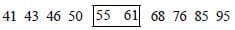Hence the median is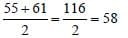SSC CGL Previous Year Questions: Number System and HCF & LCM- 1 - Question 3

### What is the value of x so that the seven digit number 8439 x 53 is divisible by 99?       (SSC CGL-2018)

Detailed Solution for SSC CGL Previous Year Questions: Number System and HCF & LCM- 1 - Question 3

8439 x 53 is divisible by 99 i.e. given number is divisible by 11
∴ (3 + x + 3 + 8) - (5 + 9 + 4) = 0
x = 4

SSC CGL Previous Year Questions: Number System and HCF & LCM- 1 - Question 4

If the six digit number 15x1y2 is divisible by 44, then (x + y) is equal to:      (SSC CGL-2018)

Detailed Solution for SSC CGL Previous Year Questions: Number System and HCF & LCM- 1 - Question 4

Any number which divisible by 44, must be divisible by 11 also is
And for any number divisible by 11 the difference of sum of its digits at odd and even places be divisible by 11.
For the given number 15x 1y2
(x + y + 1) – (5 + 1 + 2) = 0
x + y = 7

SSC CGL Previous Year Questions: Number System and HCF & LCM- 1 - Question 5

Which among the following numbers is exactly divisible by 11,13 and 7? (SSC CHSL-2018)

Detailed Solution for SSC CGL Previous Year Questions: Number System and HCF & LCM- 1 - Question 5

L. C. M. of 11, 13 and 7
= 11 × 13 × 7 = 1001.
Now, from given option '259259' is divisible by '1001'.
Hence, '259259' is divisible by 11, 13 and 7.

SSC CGL Previous Year Questions: Number System and HCF & LCM- 1 - Question 6

If x =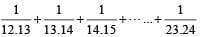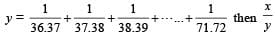is equal to :        (SSC CHSL-2018)

Detailed Solution for SSC CGL Previous Year Questions: Number System and HCF & LCM- 1 - Question 6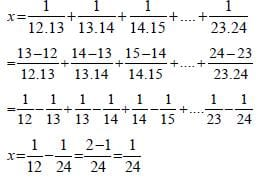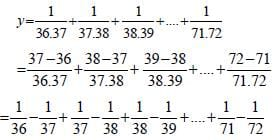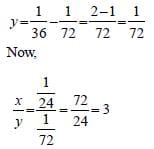SSC CGL Previous Year Questions: Number System and HCF & LCM- 1 - Question 7

The square root of which of the following is a rational number?       (SSC Sub. Ins. 2018)

Detailed Solution for SSC CGL Previous Year Questions: Number System and HCF & LCM- 1 - Question 7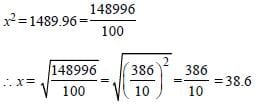SSC CGL Previous Year Questions: Number System and HCF & LCM- 1 - Question 8

Two numbers are in the ratio 4 : 7. If their HCF is 26, then the sum of these two numbers will be:       (SSC Sub. Ins. 2018)

Detailed Solution for SSC CGL Previous Year Questions: Number System and HCF & LCM- 1 - Question 8

Let the two numbers are 4x and 7x.
H. C. F. of 4x and 7x = x.
Now, x = 26.
So, two numbers are 4 × 26 and 7 × 26.
Sum of two numbers = 4 × 26 + 7 × 26 = 11 × 26 = 286.

SSC CGL Previous Year Questions: Number System and HCF & LCM- 1 - Question 9

If the seven digit number 74x29y6 is divisible by 72, then what will be the value of (2x + 3y)?       (SSC Sub. Ins. 2018)

Detailed Solution for SSC CGL Previous Year Questions: Number System and HCF & LCM- 1 - Question 9

Any number that is divisible by 72 must be divisible by 3, 4, 8, and 9.
Now, a number is divisible by 4 when a number formed by its last two digits of that number is divisible by 4. 'y6' is divisible by 4, for y = 1, 3, 5, 7, 9
Again a, number is divisible by 8, when a number formed by its last 3 digits is divisible by 8.
'9y6' is divisible by '8' for y = 3, 7
Now, for divisibility by 9, sum of its digits should be divisible by 9.
for y = 3, '7 + 4 + x + 2 + 9 + 3 + 6' = 31 + x
so, for x = 5, 36 is divisible by 9.
Now, is '7452936' which is divisible by '24' also, so, it is divisible '72'.
Now, (2x + 3y) = 2 × 5 + 3 × 3 = 19.

SSC CGL Previous Year Questions: Number System and HCF & LCM- 1 - Question 10

What is the sum of the digits of the least number, which when divided by 12,16 and 54, leaves the same remainder 7 in each case, and is also completely divisible by 13?       (SSC Sub. Ins. 2018 )

Detailed Solution for SSC CGL Previous Year Questions: Number System and HCF & LCM- 1 - Question 10

L.C.M. of 12, 16 and 54.
12 = 2 × 2 × 3.
16 = 2 × 2 × 2 × 2.
54 = 2 × 3 × 3 × 3
L.C.M. = 2 × 2 ×2 × 2 × 3 × 3 × 3 = 432
Remainder = 7.
So, required number = 432 + 7 = 439.
But this is not divisible by 13.
so, next number is 432 × 2 + 7 = 871.
Number 871 is divisible by 13.
Hence, required number is 871.
Sum of its digits = 8 + 7 + 1 = 16.

SSC CGL Previous Year Questions: Number System and HCF & LCM- 1 - Question 11

If 34N is divisible by 11, then what is the value of N?      (SSC Sub. Ins. 2017)

Detailed Solution for SSC CGL Previous Year Questions: Number System and HCF & LCM- 1 - Question 11

A number is divisible by 11, if difference of the sum of the digits at the even places and sum of digits at odd places is either 0 (zero) or a multiple of 11.
Now,
(3 + N) – 4 = 0
3 + N – 4 = 0
N – 1 = 0
∴ N = 1

SSC CGL Previous Year Questions: Number System and HCF & LCM- 1 - Question 12

Which one among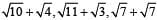is the smallest number?        (SSC Sub. Ins. 2017)

Detailed Solution for SSC CGL Previous Year Questions: Number System and HCF & LCM- 1 - Question 12

Here,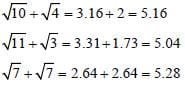So,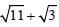is the smallest number.

SSC CGL Previous Year Questions: Number System and HCF & LCM- 1 - Question 13

What is the smallest value that must be added to 709, so that the resultant is a perfect square?        (SSC Sub. Ins. 2017)

Detailed Solution for SSC CGL Previous Year Questions: Number System and HCF & LCM- 1 - Question 13

According to question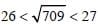Now, (27)2 = 729
∴ 729 – 709 = 20
∴ 20 must be added to 709 to make it a perfect square.

SSC CGL Previous Year Questions: Number System and HCF & LCM- 1 - Question 14

Of the three numbers, the first is twice the second, and the second is twice the third. The average of the reciprocal of the numbers is 7/12. The numbers are:        (SSC MTS 2017)

Detailed Solution for SSC CGL Previous Year Questions: Number System and HCF & LCM- 1 - Question 14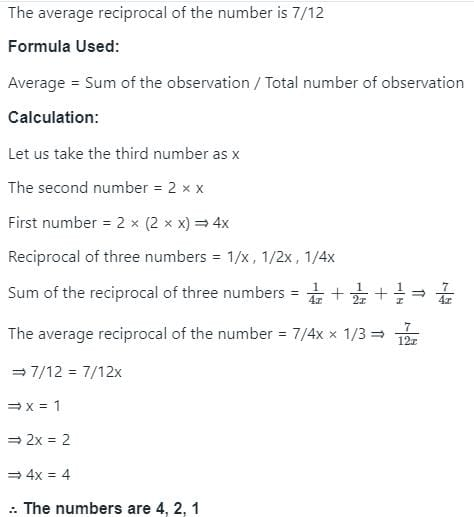SSC CGL Previous Year Questions: Number System and HCF & LCM- 1 - Question 15

The least number of five digits exactly divisible by 88 is:        (SSC MTS 2017)

Detailed Solution for SSC CGL Previous Year Questions: Number System and HCF & LCM- 1 - Question 15

The smallest number of 5 digits = 10000
Now, 10000/88 = 113, and remainder is 56
∴ Required number = 10000 + (88 – 56) = (10000 + 32) = 10032.

SSC CGL Previous Year Questions: Number System and HCF & LCM- 1 - Question 16

Product of digits of a 2–digit number is 27. If we add 54 to the number, the new number obtained is a number formed by interchange of the digits. Find the number.       (SSC CHSL 2017)

Detailed Solution for SSC CGL Previous Year Questions: Number System and HCF & LCM- 1 - Question 16

Let digit at ten's place be x and digit at unit's place be y.
∴ The number = 10x + y
When digit are interchanged, the new number
= 10y + x
According to question,
Product of digits = 27 i.e., xy = 27 ...(i)
Also,
10x+ y + 54 = 10y + x
9x – 9y = – 54
x – y = – 6
∴ x = y – 6 ...(ii)
From (i) and (ii),
y (y – 6) = 27
y2 – 6y – 27 = 0
y2 – 9y + 3y – 27 = 0
(y – 9) (y + 3) = 0
∴ y = 9 or y = – 3
∴ x = 3
When x = 3, and y = 9
∴ Required number = 10x + y
= 10 × 3 + 9
⇒ 30 + 9 = 39.

SSC CGL Previous Year Questions: Number System and HCF & LCM- 1 - Question 17

What is the LCM (least common multiple) of 57 and 93?       (SSC CHSL 2017)

Detailed Solution for SSC CGL Previous Year Questions: Number System and HCF & LCM- 1 - Question 17

LCM of 57 and 93,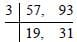⇒ 3 × 19 × 31 = 1767.

SSC CGL Previous Year Questions: Number System and HCF & LCM- 1 - Question 18

By which least number should 5000 be divided so that it becomes a perfect square?       (SSC CGL 2017)

Detailed Solution for SSC CGL Previous Year Questions: Number System and HCF & LCM- 1 - Question 18

According to option,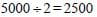Hence, 2500 is a perfect square of 50.

SSC CGL Previous Year Questions: Number System and HCF & LCM- 1 - Question 19

Which value among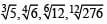is the largest?       (SSC CGL 2017)

Detailed Solution for SSC CGL Previous Year Questions: Number System and HCF & LCM- 1 - Question 19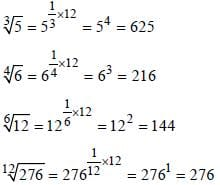SSC CGL Previous Year Questions: Number System and HCF & LCM- 1 - Question 20

How many numbers are there from 300 to 650 which are completely divisible by both 5 and 7?        (SSC CGL 2017)

Detailed Solution for SSC CGL Previous Year Questions: Number System and HCF & LCM- 1 - Question 20

LCM of 5 and 7 = 35
So, the numbers divisible by both 5 and 7 are multilpe of 35. Between 300 and 650. We have 10 multiple of 35. They are : 315, 350, 385, 420, 455, 490, 525, 560, 595, 630.

## SSC CGL (Tier - 1) - Previous Year Papers (Topic Wise)

250 tests
Information about SSC CGL Previous Year Questions: Number System and HCF & LCM- 1 Page
In this test you can find the Exam questions for SSC CGL Previous Year Questions: Number System and HCF & LCM- 1 solved & explained in the simplest way possible. Besides giving Questions and answers for SSC CGL Previous Year Questions: Number System and HCF & LCM- 1, EduRev gives you an ample number of Online tests for practice

250 tests